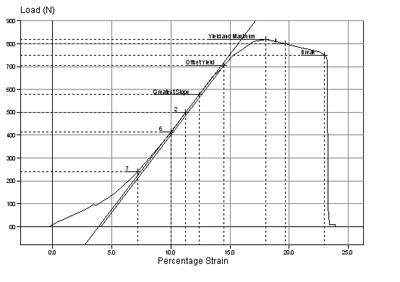## Modulus of Elasticity Testing

Posted on July 12, 2016

Modulus of elasticity (or Young’s Modulus) is a measurement of the rate of change of strain as a function of stress. It represents the slope of the straight-line portion of a stress-strain curve. With respect to tensile testing, it may be referred to as Tensile Modulus.

This method of testing is used to determine a sample’s behaviour under an axial stretching load. Common tensile test results include elastic limit, tensile strength, yield point, yield strength, elongation, and Young’s Modulus. Young’s Modulus is reported commonly as N/mm2 (lbs/in2), MPA (psi).

Typical graph showing modulus of elasticity / Young’s Modulus: